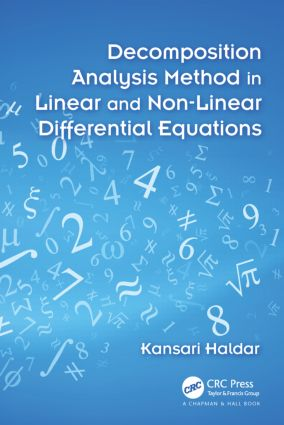# Decomposition Analysis Method in Linear and Nonlinear Differential Equations

## 1st Edition

Chapman and Hall/CRC

274 pages | 20 B/W Illus.

##### Purchasing Options:\$ = USD
Hardback: 9781498716338
pub: 2015-10-16
SAVE ~\$22.00
\$110.00
\$88.00
x
eBook (VitalSource) : 9780429157585
pub: 2015-10-22
from \$28.98

FREE Standard Shipping!

### Description

A Powerful Methodology for Solving All Types of Differential Equations

Decomposition Analysis Method in Linear and Non-Linear Differential Equations explains how the Adomian decomposition method can solve differential equations for the series solutions of fundamental problems in physics, astrophysics, chemistry, biology, medicine, and other scientific areas. This method is advantageous as it simplifies a real problem to reduce it to a mathematically tractable form.

The book covers the four classes of the decomposition method: regular/ordinary decomposition, double decomposition, modified decomposition, and asymptotic decomposition. It applies these classes to Laplace and Navier–Stokes equations in Cartesian and polar coordinates for obtaining partial solutions of the equations. Examples of physical and physiological problems, such as tidal waves in a channel, fluids between plates and through tubes, the flow of blood through arteries, and the flow past a wave-shaped wall, demonstrate the applications.

Drawing on the author’s extensive research in fluid and gas dynamics, this book shows how the powerful decomposition methodology of Adomian can solve differential equations in a way comparable to any contemporary superfast computer.

Decomposition Method

Introduction

Partial Solutions of a Partial Differential Equation

A Review on the Convergence of the Decomposition Method

Asymptotic Decomposition

Introduction

Application of Asymptotic Decomposition

Bessel’s Equation

Introduction

Solution of Bessel’s General Equation by Modified Decomposition

Second Solution of Bessel’s Equation by Regular Decomposition

Pulsatile Flow of Fluid in a Rigid Tube

Periodic Motion of a Visco-Elastic Fluid in a Rigid Tube

Tidal Waves in a Channel Open to the Sea

Temperature Distribution in an Infinitely Long Circular Cylinder

Navier-Stokes Equations in Cartesian Coordinates

Introduction

Equations of Motion

Steady Laminar Flow of Viscous Fluid through a Tube of an Elliptic Cross Section

Stokes’s First Problem: The Suddenly Accelerated Plane Wall

Stokes’s Second Problem: The Flow Near an Oscillating Flat Plate

Unsteady Flow of Viscous Incompressible Fluid between Two Parallel Plates

Pulsatile Flow between Two Parallel Plates

Navier-Stokes Equations in Cylindrical Polar Coordinates

Introduction

Equations of Motion

Hagen-Poiseuille Theory: The Steady Laminar Flow of Fluid through a Circular Tube

Couette Flow: Steady Laminar Flow between Two Concentric Rotating Circular Cylinders

Flow in Convergent and Divergent Channels

Blood Flow in Artery

Introduction

Steady Flow of Blood through a Constricted Artery

Flow of Blood through Arteries in the Presence of a Magnetic Field

Pulsatile Flow of Blood through a Constricted Artery

Introduction

Equations of Motion

Application of Regular Decomposition to a Linearized Gasdynamic Equation for Plane Flow

Application of Modified Decomposition to a Linearized Gasdynamic Equation for Plane Flow

Flow Past a Wavy Wall

Application of Regular Decomposition to a Linearized Gasdynamic Equation for Axisymmetric Flow

Flow Past a Corrugated Circular Cylinder

Introduction

Transonic Solution by Regular Decomposition

Transonic Solution by Modified Decomposition

Transonic Solution by Multidimensional Operator

Transonic Flow Past a Wavy Wall

Laplace’s Equation

Introduction

Solution of Laplace’s Equation by Regular Decomposition

Solution of Laplace’s Equation by Modified Decomposition

Laplace’s Equation for a Circular Disc

Laplace’s Equation for a Circular Annulus

Flow Near a Rotating Disc in a Fluid at Rest

Introduction

Equations of Motion

Solutions for the Small Value of η

Solutions for the Large Value of η

Appendix

Index

References appear at the end of each chapter.

### About the Author

Kansari Haldar retired as a professor from the Indian Statistical Institute, Kolkata. His work has spanned 35 years, covering fluid dynamics, gas dynamics, hydrodynamics, biofluid dynamics, biomagnetofluid dynamics, and Adomian’s decomposition methodology.

### Subject Categories

##### BISAC Subject Codes/Headings:
MAT003000
MATHEMATICS / Applied
MAT007000
MATHEMATICS / Differential Equations
MAT021000
MATHEMATICS / Number Systems